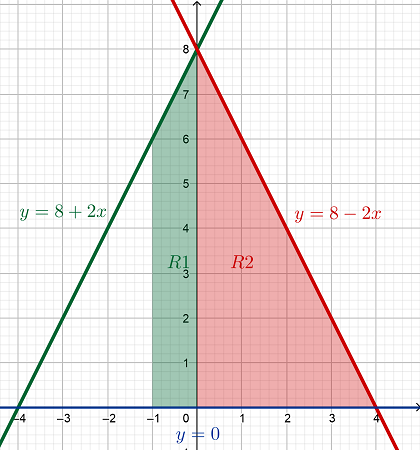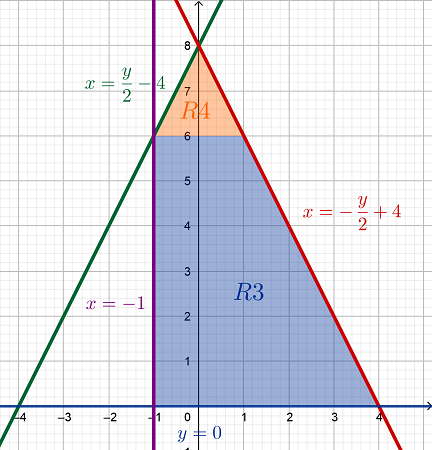# Reverse the order of the integration in the following expression \int_{-1}^0 \int_0^{8+2x} Q dy...

## Question:

Reverse the order of the integration in the following expression

{eq}\displaystyle \int_{-1}^0 \int_0^{8+2x} Q dy dx +\int_0^4 \int_0^{8-2x}Q dy dx {/eq}

where Q is an unknown function of x and y

## Evaluating Double Integrals using Iterated Integrals:

Consider the integral of the function {eq}F(x,y) {/eq} over the planar region {eq}D {/eq} on the xy-plane, expressed as:

$$\iint \limits_D F(x,y) \,dA$$

Suppose the region of integration is a Type I region, one which is bounded by continuous functions of x, such that {eq}D = \left\{\, (x,y) \,|\, a \le x \le b \text{ and } g_1(x) \le y \le g_2(x) \,\right\} {/eq}.

Then, the double integrals above can be written as the iterated integrals:

$$\iint \limits_D F(x,y) \,dA = \int_a^b \int_{g_1(x)}^{g_2(x)} F(x,y) \,dy \,dx$$

On the other hand, suppose the region of integration is a Type II region, one which is bounded instead by continuous functions of y, such that {eq}D = \left\{\, (x,y) \,|\, c \le y \le d \text{ and } h_1(y) \le x \le h_2(y) \,\right\} {/eq}.

Then, the double integrals above can also be written as the iterated integrals:

$$\iint \limits_D F(x,y) \,dA = \int_c^d \int_{h_1(y)}^{h_2(y)} F(x,y) \,dx \,dy$$

Given the sum of the two iterated integrals {eq}\begin{align*} \int_{-1}^0 \int_0^{8 + 2x} Q(x,y) \,dy \,dx + \int_0^4 \int_0^{8 - 2x} Q(x,y) \,dy \,dx \end{align*} {/eq}

Let {eq}R1 \text{ and } R2 {/eq} be the regions of integration of the first and second iterated integrals, respectively. Looking at the order of integration, {eq}dy \,dx {/eq}, these two regions are defined as Type I regions.

Looking at the limits of integration:

• Region {eq}R1 {/eq} is bounded above by {eq}y = 8 + 2x {/eq} and below by {eq}y = 0 {/eq} on the interval {eq}-1 \le x \le 0 {/eq}; and,
• Region {eq}R2 {/eq} is bounded above by {eq}y = 8 - 2x {/eq} and below by {eq}y = 0 {/eq} on the interval {eq}0 \le x \le 4 {/eq}.To reverse the order of integration, the union of the two regions {eq}R1 \text{ and } R2 {/eq}, which represents the whole region of integration, must be defined as Type II region/s.

This can be done by dividing the region of integration into two smaller regions using the horizontal line {eq}y = 6 {/eq}. Let {eq}R3 \text{ and } R4 {/eq} be the resulting smaller Type II regions.

Convert the equations of the diagonal lines into functions of y:

{eq}\begin{align*} &y = 8 + 2x \\ &\Rightarrow 2x = y - 8 \\ &\Rightarrow x = \frac{y}{2} - 4 & \text{[Left Diagonal Line (green)]} \\ \\ &y = 8 - 2x \\ &\Rightarrow 2x = 8 - y \\ &\Rightarrow x = - \frac{y}{2} + 4 & \text{[Right Diagonal Line (red)]} \end{align*} {/eq}

Then, based on the graph and the functions above, the two Type II regions can be defined as {eq}R3 = \left\{\, (x,y) \,|\, 0 \le y \le 6 \text{ and } -1 \le x \le - \frac{y}{2} + 4 \,\right\} \text{ and } R4 = \left\{\, (x,y) \,|\, 6 \le y \le 8 \text{ and } \frac{y}{2} - 4 \le x \le - \frac{y}{2} + 4 \,\right\} {/eq}.Therefore, the given iterated integrals when the order of integration is reversed are equal to:

{eq}\begin{align*} &\int_{-1}^0 \int_0^{8 + 2x} Q(x,y) \,dy \,dx + \int_0^4 \int_0^{8 - 2x} Q(x,y) \,dy \,dx = \iint \limits_{R3} Q(x,y) \,dA + \iint \limits_{R4} Q(x,y) \,dA \\ &\boxed{ \int_{-1}^0 \int_0^{8 + 2x} Q(x,y) \,dy \,dx + \int_0^4 \int_0^{8 - 2x} Q(x,y) \,dy \,dx = \int_0^6 \int_{-1}^{-\frac{y}{2}+4} Q(x,y) \,dx \,dy + \int_6^8 \int_{\frac{y}{2}-4}^{-\frac{y}{2}+4} Q(x,y) \,dx \,dy } \end{align*} {/eq}